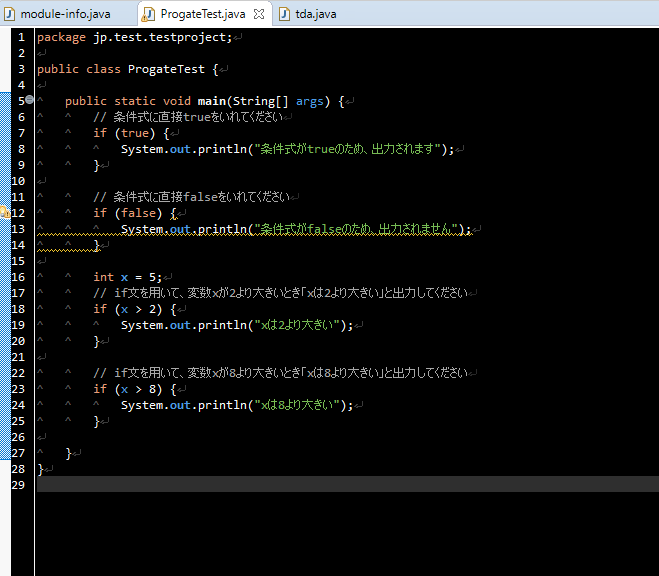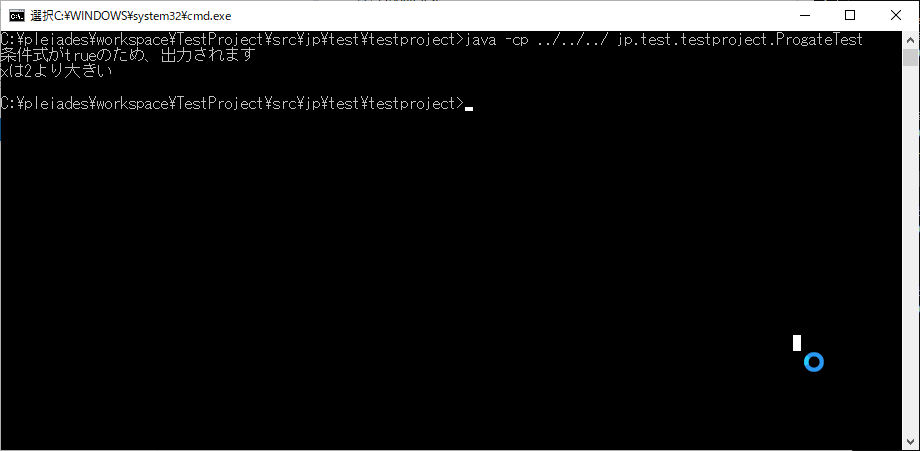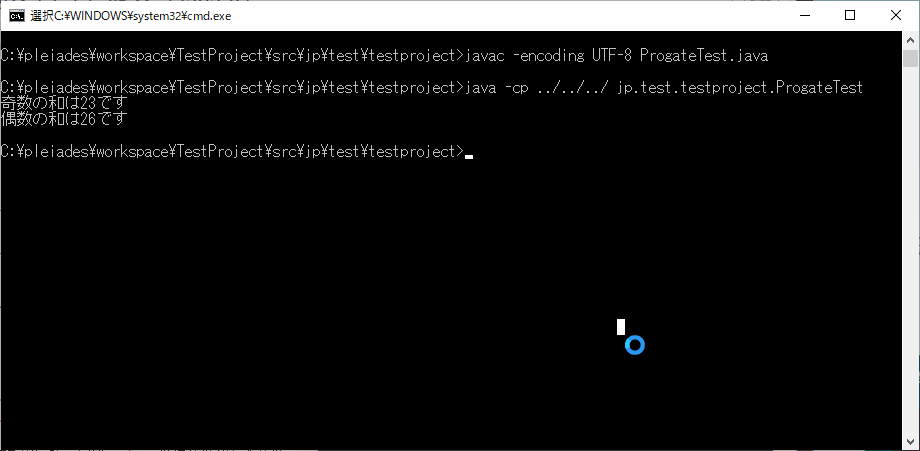# Try the free version of Progate [Java II]

Continuing from Last time, I will continue to do Progate free lessons.

This time it's Java II. This is free.

Lesson List

Java II

Official lesson

### Logical operator

・ Because it is a basic thing ... omitted

### if statement

・ Basically ... I have to put a little on it ...Where there is a wavy line, it is a "dead code". As the name implies, it is false, so the processing inside the if block is never executed.

Execution result from command promptelse, else if ・ Java is written as ʻelse if`. ~~ It feels better than ʻelsif` ... ~~ As pointed out in another article, ʻelse if` is not a single conditional branch, It seems that ʻelse` and ʻif` are consecutive and they are only written in one line.

``````//this
if (x == 8) {
System.out.println("8");
} else {
if (x > 8) {
System.out.println("Greater than 8");
} else {
System.out.println("Less than 8");
}
}

//↓ else as shown below{}Just abbreviated

if (x == 8) {
System.out.println("8");
} else if (x > 8) {
System.out.println("Greater than 8");
} else {
System.out.println("Less than 8");
}

//* I don't have the indentation.
``````

So I understood that it is different from Ruby's ʻelif` and Python's ʻelif`. The result of the process will be the same.

By the way, for java, it is better to use ʻequals` for string comparison.

``````var test = new String("test");
System.out.println(test == "test"); //Become false

System.out.println(test.equals("test")); //Become true
``````

`==` is comparing the reference destination of the stored memory. ① `var test = new String ("test") `is first stored in memory. ② `System.out.println (test ==" test ");` "test" `is stored in a place different from ①. Therefore, it is judged to be different.

So

``````var test = new String("test");
var test2 = test;
System.out.println(test == test2 ); //Will be true.
``````

This will be true. Because the reference destination is the same.

Also, it's really complicated,

``````var test = "test";
System.out.println(test == "test"); //Become true
``````

Will be true. Apparently, if you set the literal `" test "`, it will refer to the same place if it is the same character string. It's complicated ...

For the time being, if you are using ʻequals`, you can make a mistake.

### switch statement / switch statement (2)

・ Basic things. `case``default``break;`

### while statement / for statement

・ Java for is also orthodox

``````for (var i = 0; i < count; i++) {
}
``````

Form of. If it's a lesson, I get angry when I use var ...

break, continue ・ Break out of the break loop. ・ Continue skip, next element.

### Let's use an array

``````new String[] {"test","bbb"};
``````

Initialization.

``````{"test", "bbb"};
``````

But it looks like I can go ... I did not know···. C # is probably impossible ...

### Overwriting the elements of the array, repeating the array

·nothing special···.

### Extended for statement

・ Foreach in C #

``````var numbers2 = new int[]{1, 2, 3, 4};
for (var number2 : numbers2) {
}
``````

** Exercise **

``````package jp.test.testproject;

public class ProgateTest {

public static void main(String[] args) {
//Substitute the given array of numbers for the variable numbers
int[] numbers = {1, 4, 6, 9, 13, 16};

int oddSum = 0;
int evenSum = 0;

//Use the for statement to find the sum of even and odd numbers in the array numbers
for (int number : numbers) {
if(number % 2 == 0) {
evenSum += number;
} else {
oddSum += number;
}
}

System.out.println("The sum of odd numbers is" + oddSum + "is");
System.out.println("The sum of even numbers" + evenSum + "is");
}
}
``````

result** Cleared **# Impressions

It's terrible ... It's an article with almost no content ... It's too basic, and the introductory editions of other languages that I've done so far are enough.

Next time, I would like to do Python I.